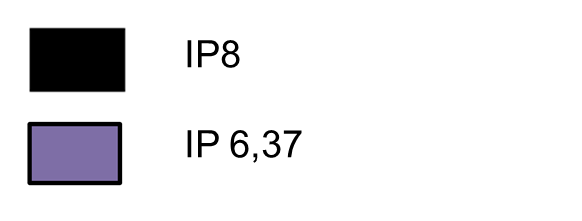# Problems between IP 6.37 and 8.0Good morning, everyone,

I'm new to IP 8. On my IP 6.37, it is possible to make a legend with a colored trace. The color is the same as the fill color but on IP 8.0 the color is the same as the line color (see attachement). Do you have any suggestions for doing the same thing on IP 8?

Thank you

ThomasOf course, I have a macro to adapt the XPS data and at the output I want to trace the peaks with this loop. And I don't have the same result with the different IP versions as the one indicated in the last message.

Function Plot(Source)
Wave Source

String Legends=""
Variable i
Display Source
for (i = 0; i <ItemsInList(WaveList("p*",";","")); i += 1)     // set new color offset
AppendToGraph \$StringFromList(i,WaveList("p*",";",""))
ModifyGraph mode(\$StringFromList(i,WaveList("p*",";","")))=7
ModifyGraph toMode(\$StringFromList(i,WaveList("p*",";","")))=1
ModifyGraph hbFill(\$StringFromList(i,WaveList("p*",";","")))=2
ModifyGraph lsize(\$StringFromList(i,WaveList("p*",";","")))=2
ModifyGraph usePlusRGB(\$StringFromList(i,WaveList("p*",";","")))=1
ModifyGraph rgb(\$StringFromList(i,WaveList("p*",";","")))=(0,0,0)
ModifyGraph useNegPat=1
Legends=Legends+"\\s("+StringFromList(i,WaveList("p*",";",""))+")\r"
endfor

End

Thomas

Disclaimer: I don't have IP6 at hand (only 8 and 7) but if you execute the following

`Make/O/N=20 source=p, p1=p+2+enoise(1), p2 = p+5+enoise(2)`

and then call

`plot1(source)`

Function Plot1(Source)
Wave Source
String ThisTrace
Variable i
Display source
Legend
for (i = 0; i <ItemsInList(WaveList("p*",";","")); i += 1)     // set new color offset
ThisTrace = StringFromList(i,WaveList("p*",";",""))
AppendToGraph \$ThisTrace
ModifyGraph mode(\$ThisTrace)=7
ModifyGraph toMode(\$ThisTrace)=1
ModifyGraph hbFill(\$ThisTrace)=2
ModifyGraph lsize(\$ThisTrace)=2
ModifyGraph usePlusRGB(\$ThisTrace)=1
ModifyGraph rgb(\$ThisTrace)=(0,0,0)
ModifyGraph useNegPat=1
endfor
End

Do you really get something different?

Using the executable example provided by ChrLie on Macintosh, I do see a difference between Igor6 and Igor8. In Igor6 the p1 and p2 legend boxes are filled with red while in Igor8 they are filled with black. This may be a bug in Igor8. We will investigate.

BTW: Providing an executable example, whether in the form of commands or an experiment file, is the quickest way to get to a resolution. For graph problems it is often helpful to export the graph as an experiment file using File-> Save Graph Copy which you can post here or send to WaveMetrics support.

In the short term, you can use \s(traceName) instead of just \s(traceName) to force Igor 7 and 8 to draw the +Fill Color as the symbol for the entire trace.

Igor 8.05 and Igor 9 will restore the Igor 6 behavior you've come to expect.ForumSupportGallery